#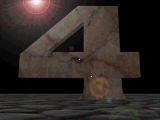Four

4 is a number, a word or symbol used to designate quantities or, by extension, entities having quantitylike properties.

### Rational Numbers

The simplest numbers are the natural numbers, 1, 2, 3, ..., used in counting; they are also called the whole numbers, positive integers, or positive rational integers. The natural numbers are closed with respect to addition and multiplication; that is, the sum and product of two natural numbers are always natural numbers. Because the quotient of two natural numbers, however, is not always a natural number, it is convenient to introduce the positive fractions to represent the quotient of any two natural numbers. The natural number n is identified with the fraction n/1. Furthermore, because the difference of two positive fractions is not always a positive fraction, it is expeditious to introduce the negative fractions (including the negative integers) and the number zero (0). The positive and negative integers and fractions, and the number 0, comprise the rational number system.

The sum, difference, product, or quotient of two rational numbers is always a rational number. Division of any number by zero, however, is not allowed. It can be shown that every rational number can be represented as a repeating or periodic decimal, that is, as a number in the decimal notation, which after a certain point consists of the infinite repetition of a finite block of digits. Conversely, every repeating decimal represents a rational number. Thus, 617/50 = 12.34000 ..., and 2317/990 = 2.34040 ... The first expression is usually written as 12.34, omitting the infinite repetition of the block consisting of the single digit 0. The second expression is frequently written as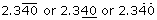to indicate that the block of two digits, 4 and 0, is repeated infinitely. The first type of expression is called a finite or terminating decimal, and the second type of expression is called an infinite periodic or repeating decimal (the term infinite is frequently omitted).

### Irrational Numbers

The development of geometry indicated the need for more numbers; the length of the diagonal of a square with sides one unit long cannot be expressed as a rational number. Similarly, the ratio of the circumference to the diameter of a circle is not a rational number. These and other needs led to the introduction of the irrational numbers. A decimal expansion that is neither of the two types described above represents an irrational number. For example,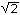= 1.4142135623 ... and= 3.1415926535 ... are irrational numbers, and their decimal expansions are necessarily nonterminating and nonperiodic. The totality of the rational and irrational numbers makes up the so-called real number system.

### Imaginary Numbers

The product of a real number multiplied by itself is 0 or positive; the equation x= -1 has no solutions in the real number system. If such a solution is desired, new numbers must be invented. Let i =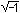be a new number representing a solution of the preceding equation. All numbers of the form a + bi, in which a and b are real numbers, belong to the complex number system. If b is not 0, the complex number is called an imaginary number; if b is not 0 but a is 0, the complex number is called a pure imaginary; if b is 0, the complex number is a real number.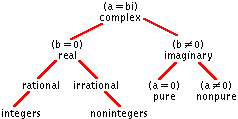Imaginary numbers (the term must not be used in a literal sense but in the technical sense just described) are extremely useful in the theory of alternating currents and many other branches of physics and natural science.

### Complex Numbers

In 1799 the German mathematician Carl Friedrich Gauss proved that every algebraic equation of degree n having the form x+ ax+ ... + ak+ a= 0 in which a, a..., aare arbitrary complex numbers, is satisfied by at least one complex root.

Whereas real numbers represent points on a line, complex numbers can be placed in correspondence with the points on a plane. To represent the complex number a + bi geometrically, the x-axis is used as the axis of the real number a, and the y-axis serves as the axis of the pure imaginary bi; the complex number, therefore, corresponds to the point P with the rectangular coordinates a and b. The line, or vector, joining the origin with the point P (a,b) is the diagonal of a rectangle with the sides a and bi.

If the complex number a + bi is multiplied by -1, the vector OP is rotated through 180 degrees, and the point P falls in the third quadrant; a rotation of 90 degrees, therefore, represents multiplication of the complex number by i, as -1 = 1, and i(a + bi) represents a point in the second quadrant if a + bi represents a point in the first.

Mystical and magical qualities have been ascribed to numbers both in antiquity and in modern times. The pseudoscience of numerology attempts to interpret the occult by means of the symbolism of numbers, which is based on the Pythagorean doctrine that all things are numbers and consist of geometrical figures in various patterns.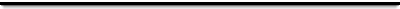Fun Facts about the number Four: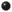It's 2+2, 2*2, and 2!It's the first non-prime numberIt is the number of knees possessed by only one species on Earth: The Elephant.